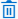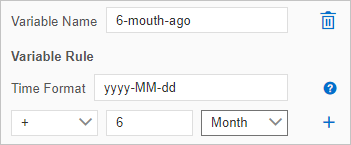This topic describes the four types of variables involved in task orchestration and shows you how to configure time variables.

Overview

• Node variables are time variables that can be used on the current node. Node variables are not displayed in the input variables of downstream nodes. Node variables are invisible to and cannot be referenced by downstream nodes.
• Task flow variables are time variables that can be used on all nodes of the current task flow. Task flow variables are displayed in the input variables of downstream nodes. Task flow variables are visible to and can be referenced by downstream nodes.
• Input variables include task flow variables and the output variables of upstream nodes. You can use \${Variable name} to reference an input variable in the SQL statement for the current node, such as an SQL Assignment for Single Instance node. You can also use an input variable as a filter condition for the current node, such as a Conditional Branch node.
• Output variables are defined and have their values assigned on the current node. Output variables can be accessed and referenced by downstream nodes. You can view the output variables of the current node in the input variables of its downstream nodes.

For example, you can use the SQL statements for value assignment to read table data in a database. Then, you can configure output variables to pass the variable values to downstream nodes.

Configure time variables

Parameter Description
Variable Name The name of the custom time variable.
Note To delete a time variable that you configured, click theicon to the right of the Variable Name field.
Variable Rule The time format and time offset configurations of the custom time variable.
• Time Format: the time format of the time variable. For more information about time formats, see the Time formats section of this topic.
• Time offset: Time variables are offset from the data timestamp, which is one day before the current date. In other words, the value of a custom time variable indicates the date to which the specified time offset points based on the data timestamp.

For example, you have created a variable named 6_month_ago in the yyyy-MM-dd format and set the offset to "- 6 Month". In this case, if the current date is August 12, 2021, the value of the \${6_month_ago} variable indicates February 11, 2021.Note After you configure a time variable, you can reference the variable in the \${Variable name} format in the SQL statement that you enter in the SQL section to the right of the Variable Setting tab. You can also click SQLPreview to view the value of the time variable.

Time formats

The following table describes the time variables that can be used to define the time format of a custom variable.

Time variable Description Sample format Sample value
Year
• y or yyyy in the four-digit format indicates the year of the day when the task is run.
• yy indicates the last two digits of the year.
• Y: the year of the last day in the week when the task is run. The last day of the week is Sunday.
yyyy 2021
Month M: the month of the year when the task is run. Valid values of M: [1,12]. Valid values of MM: [01,12]. MM 08
Week
• w: the week of the year when the task is run. Valid values of w: [1,52]. Valid values of ww: [01,52].
• W: the week of the month when the task is run. Valid values: [1,5].
ww 13
Day
• D: the day of the year when the task is run. Valid values of D: [1,365]. Valid values of DD: [01,365]. Valid values of DDD: [001,365].
• d: the day of the month when the task is run. Valid values of d: [1,31]. Valid values of dd: [01,31].
D 360
Day of the week
• E: the day of the week when the task is run. Valid values: Monday to Sunday.
• e: the number of the day in the week when the task is run. Valid values: [1,7]. A value of 1 indicates Monday.
Note To count Sunday as the first day of the week, you can set the offset to "+ 1 Day".
e 1
Ante meridiem (AM) or post meridiem (PM) a: indicates whether the point in time when the task is run is before or after the midday. If the point in time is in the range of 00:00 to 11:59, the return value is AM. If the point in time is in the range of 12:00 to 23:59, the return value is PM. a AM
Hour
• H: the hour of the day when the task is run. A value of 0 indicates the first hour of the day. Valid values of H: [0,23]. Valid values of HH: [00,23].
• h: the hour of the half day when the task is run. A value of 1 indicates the first hour of the half day. Valid values of h: [1,12]. Valid values of hh: [01,12].
• K: the hour of the half day when the task is run. A value of 0 indicates the first hour of the half day. Valid values of K: [0,11]. Valid values of KK: [00,11].
• k: the hour of the day when the task is run. A value of 1 indicates the first hour of the day. Valid values of k: [1,24]. Valid values of kk: [01,24].
HH 10
Minute m: the minute of the hour when the task is run. Valid values of m: [0,59]. Valid values of mm: [00,59]. m 27
Second
• s: the second of the minute when the task is run.
• S: the millisecond of the minute when the task is run.
ss 08
Time zone z: the time zone. z UTC+08:00

The following table describes the sample time formats that use multiple time variables.

Sample format Sample value
yyyy-MM-dd 2021-08-12
yyyyMM01 20210801
HH:mm:ss 11:05:21
yyyyMMdd HH:mm:ss 20210812 11:05:21